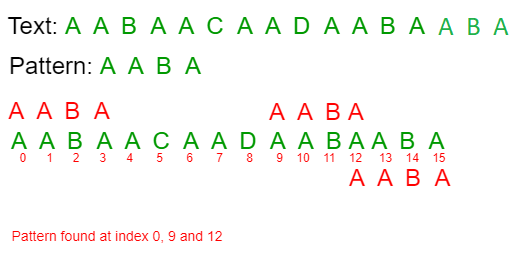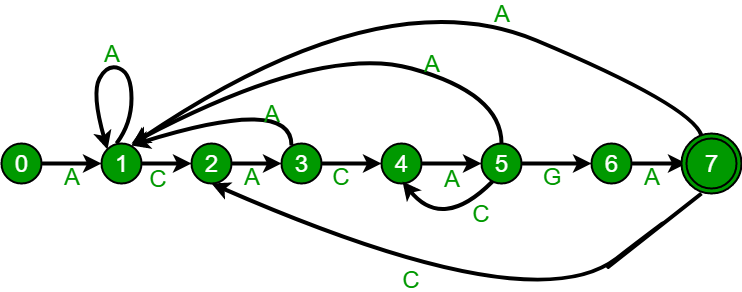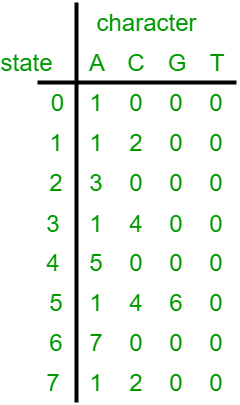Related Articles

# Finite Automata algorithm for Pattern Searching

• Difficulty Level : Hard
• Last Updated : 19 Jul, 2021

Given a text txt[0..n-1] and a pattern pat[0..m-1], write a function search(char pat[], char txt[]) that prints all occurrences of pat[] in txt[]. You may assume that n > m.
Examples:

```Input:  txt[] = "THIS IS A TEST TEXT"
pat[] = "TEST"
Output: Pattern found at index 10

pat[] =  "AABA"
Output: Pattern found at index 0
Pattern found at index 9
Pattern found at index 12```` `

Pattern searching is an important problem in computer science. When we do search for a string in notepad/word file or browser or database, pattern searching algorithms are used to show the search results.
We have discussed the following algorithms in the previous posts:
Naive Algorithm
KMP Algorithm
Rabin Karp Algorithm
In this post, we will discuss Finite Automata (FA) based pattern searching algorithm. In FA based algorithm, we preprocess the pattern and build a 2D array that represents a Finite Automata. Construction of the FA is the main tricky part of this algorithm. Once the FA is built, the searching is simple. In search, we simply need to start from the first state of the automata and the first character of the text. At every step, we consider next character of text, look for the next state in the built FA and move to a new state. If we reach the final state, then the pattern is found in the text. The time complexity of the search process is O(n).
Before we discuss FA construction, let us take a look at the following FA for pattern ACACAGA.The above diagrams represent graphical and tabular representations of pattern ACACAGA.
Number of states in FA will be M+1 where M is length of the pattern. The main thing to construct FA is to get the next state from the current state for every possible character. Given a character x and a state k, we can get the next state by considering the string “pat[0..k-1]x” which is basically concatenation of pattern characters pat, pat … pat[k-1] and the character x. The idea is to get length of the longest prefix of the given pattern such that the prefix is also suffix of “pat[0..k-1]x”. The value of length gives us the next state. For example, let us see how to get the next state from current state 5 and character ‘C’ in the above diagram. We need to consider the string, “pat[0..4]C” which is “ACACAC”. The length of the longest prefix of the pattern such that the prefix is suffix of “ACACAC”is 4 (“ACAC”). So the next state (from state 5) is 4 for character ‘C’.
In the following code, computeTF() constructs the FA. The time complexity of the computeTF() is O(m^3*NO_OF_CHARS) where m is length of the pattern and NO_OF_CHARS is size of alphabet (total number of possible characters in pattern and text). The implementation tries all possible prefixes starting from the longest possible that can be a suffix of “pat[0..k-1]x”. There are better implementations to construct FA in O(m*NO_OF_CHARS) (Hint: we can use something like lps array construction in KMP algorithm). We have covered the better implementation in our next post on pattern searching.

## C

 `// C program for Finite Automata Pattern searching``// Algorithm``#include``#include``#define NO_OF_CHARS 256` `int` `getNextState(``char` `*pat, ``int` `M, ``int` `state, ``int` `x)``{``    ``// If the character c is same as next character``    ``// in pattern,then simply increment state``    ``if` `(state < M && x == pat[state])``        ``return` `state+1;` `    ``// ns stores the result which is next state``    ``int` `ns, i;` `    ``// ns finally contains the longest prefix``    ``// which is also suffix in "pat[0..state-1]c"` `    ``// Start from the largest possible value``    ``// and stop when you find a prefix which``    ``// is also suffix``    ``for` `(ns = state; ns > 0; ns--)``    ``{``        ``if` `(pat[ns-1] == x)``        ``{``            ``for` `(i = 0; i < ns-1; i++)``                ``if` `(pat[i] != pat[state-ns+1+i])``                    ``break``;``            ``if` `(i == ns-1)``                ``return` `ns;``        ``}``    ``}` `    ``return` `0;``}` `/* This function builds the TF table which represents4``    ``Finite Automata for a given pattern */``void` `computeTF(``char` `*pat, ``int` `M, ``int` `TF[][NO_OF_CHARS])``{``    ``int` `state, x;``    ``for` `(state = 0; state <= M; ++state)``        ``for` `(x = 0; x < NO_OF_CHARS; ++x)``            ``TF[state][x] = getNextState(pat, M, state, x);``}` `/* Prints all occurrences of pat in txt */``void` `search(``char` `*pat, ``char` `*txt)``{``    ``int` `M = ``strlen``(pat);``    ``int` `N = ``strlen``(txt);` `    ``int` `TF[M+1][NO_OF_CHARS];` `    ``computeTF(pat, M, TF);` `    ``// Process txt over FA.``    ``int` `i, state=0;``    ``for` `(i = 0; i < N; i++)``    ``{``        ``state = TF[state][txt[i]];``        ``if` `(state == M)``            ``printf` `(``"\n Pattern found at index %d"``,``                                           ``i-M+1);``    ``}``}` `// Driver program to test above function``int` `main()``{``    ``char` `*txt = ``"AABAACAADAABAAABAA"``;``    ``char` `*pat = ``"AABA"``;``    ``search(pat, txt);``    ``return` `0;``}`

## CPP

 `// CPP program for Finite Automata Pattern searching``// Algorithm``#include ``using` `namespace` `std;``#define NO_OF_CHARS 256` `int` `getNextState(string pat, ``int` `M, ``int` `state, ``int` `x)``{``    ``// If the character c is same as next character``    ``// in pattern,then simply increment state``    ``if` `(state < M && x == pat[state])``        ``return` `state+1;` `    ``// ns stores the result which is next state``    ``int` `ns, i;` `    ``// ns finally contains the longest prefix``    ``// which is also suffix in "pat[0..state-1]c"` `    ``// Start from the largest possible value``    ``// and stop when you find a prefix which``    ``// is also suffix``    ``for` `(ns = state; ns > 0; ns--)``    ``{``        ``if` `(pat[ns-1] == x)``        ``{``            ``for` `(i = 0; i < ns-1; i++)``                ``if` `(pat[i] != pat[state-ns+1+i])``                    ``break``;``            ``if` `(i == ns-1)``                ``return` `ns;``        ``}``    ``}` `    ``return` `0;``}` `/* This function builds the TF table which represents4``    ``Finite Automata for a given pattern */``void` `computeTF(string pat, ``int` `M, ``int` `TF[][NO_OF_CHARS])``{``    ``int` `state, x;``    ``for` `(state = 0; state <= M; ++state)``        ``for` `(x = 0; x < NO_OF_CHARS; ++x)``            ``TF[state][x] = getNextState(pat, M, state, x);``}` `/* Prints all occurrences of pat in txt */``void` `search(string pat, string txt)``{``    ``int` `M = pat.size();``    ``int` `N = txt.size();` `    ``int` `TF[M+1][NO_OF_CHARS];` `    ``computeTF(pat, M, TF);` `    ``// Process txt over FA.``    ``int` `i, state=0;``    ``for` `(i = 0; i < N; i++)``    ``{``        ``state = TF[state][txt[i]];``        ``if` `(state == M)``            ``cout<<``" Pattern found at index "``<< i-M+1<

## Java

 `// Java program for Finite Automata Pattern``// searching Algorithm``class` `GFG {``    ` `    ``static` `int` `NO_OF_CHARS = ``256``;``    ``static` `int` `getNextState(``char``[] pat, ``int` `M, ``                             ``int` `state, ``int` `x)``    ``{``        ` `        ``// If the character c is same as next``        ``// character in pattern,then simply``        ``// increment state``        ``if``(state < M && x == pat[state])``            ``return` `state + ``1``;``            ` `        ``// ns stores the result which is next state``        ``int` `ns, i;` `        ``// ns finally contains the longest prefix``        ``// which is also suffix in "pat[0..state-1]c"` `        ``// Start from the largest possible value``        ``// and stop when you find a prefix which``        ``// is also suffix``        ``for` `(ns = state; ns > ``0``; ns--)``        ``{``            ``if` `(pat[ns-``1``] == x)``            ``{``                ``for` `(i = ``0``; i < ns-``1``; i++)``                    ``if` `(pat[i] != pat[state-ns+``1``+i])``                        ``break``;``                    ``if` `(i == ns-``1``)``                        ``return` `ns;``            ``}``        ``}` `            ``return` `0``;``    ``}` `    ``/* This function builds the TF table which``    ``represents Finite Automata for a given pattern */``    ``static` `void` `computeTF(``char``[] pat, ``int` `M, ``int` `TF[][])``    ``{``        ``int` `state, x;``        ``for` `(state = ``0``; state <= M; ++state)``            ``for` `(x = ``0``; x < NO_OF_CHARS; ++x)``                ``TF[state][x] = getNextState(pat, M, state, x);``    ``}` `    ``/* Prints all occurrences of pat in txt */``    ``static` `void` `search(``char``[] pat, ``char``[] txt)``    ``{``        ``int` `M = pat.length;``        ``int` `N = txt.length;` `        ``int``[][] TF = ``new` `int``[M+``1``][NO_OF_CHARS];` `        ``computeTF(pat, M, TF);` `        ``// Process txt over FA.``        ``int` `i, state = ``0``;``        ``for` `(i = ``0``; i < N; i++)``        ``{``            ``state = TF[state][txt[i]];``            ``if` `(state == M)``                ``System.out.println(``"Pattern found "``                          ``+ ``"at index "` `+ (i-M+``1``));``        ``}``    ``}` `    ``// Driver code``    ``public` `static` `void` `main(String[] args)``    ``{``        ``char``[] pat = ``"AABAACAADAABAAABAA"``.toCharArray();``        ``char``[] txt = ``"AABA"``.toCharArray();``        ``search(txt,pat);``    ``}``}` `// This code is contributed by debjitdbb.`

## Python

 `# Python program for Finite Automata``# Pattern searching Algorithm` `NO_OF_CHARS ``=` `256` `def` `getNextState(pat, M, state, x):``    ``'''``    ``calculate the next state``    ``'''` `    ``# If the character c is same as next character``      ``# in pattern, then simply increment state` `    ``if` `state < M ``and` `x ``=``=` `ord``(pat[state]):``        ``return` `state``+``1` `    ``i``=``0``    ``# ns stores the result which is next state` `    ``# ns finally contains the longest prefix``     ``# which is also suffix in "pat[0..state-1]c"` `     ``# Start from the largest possible value and``      ``# stop when you find a prefix which is also suffix``    ``for` `ns ``in` `range``(state,``0``,``-``1``):``        ``if` `ord``(pat[ns``-``1``]) ``=``=` `x:``            ``while``(i

## C#

 `// C# program for Finite Automata Pattern``// searching Algorithm``using` `System;` `class` `GFG``{` `public` `static` `int` `NO_OF_CHARS = 256;``public` `static` `int` `getNextState(``char``[] pat, ``int` `M,``                               ``int` `state, ``int` `x)``{` `    ``// If the character c is same as next``    ``// character in pattern,then simply``    ``// increment state``    ``if` `(state < M && (``char``)x == pat[state])``    ``{``        ``return` `state + 1;``    ``}` `    ``// ns stores the result``    ``// which is next state``    ``int` `ns, i;` `    ``// ns finally contains the longest``    ``// prefix which is also suffix in``    ``// "pat[0..state-1]c"` `    ``// Start from the largest possible ``    ``// value and stop when you find a``    ``// prefix which is also suffix``    ``for` `(ns = state; ns > 0; ns--)``    ``{``        ``if` `(pat[ns - 1] == (``char``)x)``        ``{``            ``for` `(i = 0; i < ns - 1; i++)``            ``{``                ``if` `(pat[i] != pat[state - ns + 1 + i])``                ``{``                    ``break``;``                ``}``            ``}``                ``if` `(i == ns - 1)``                ``{``                    ``return` `ns;``                ``}``        ``}``    ``}` `        ``return` `0;``}` `/* This function builds the TF table which``represents Finite Automata for a given pattern */``public` `static` `void` `computeTF(``char``[] pat,``                             ``int` `M, ``int``[][] TF)``{``    ``int` `state, x;``    ``for` `(state = 0; state <= M; ++state)``    ``{``        ``for` `(x = 0; x < NO_OF_CHARS; ++x)``        ``{``            ``TF[state][x] = getNextState(pat, M,``                                        ``state, x);``        ``}``    ``}``}` `/* Prints all occurrences of``   ``pat in txt */``public` `static` `void` `search(``char``[] pat,``                          ``char``[] txt)``{``    ``int` `M = pat.Length;``    ``int` `N = txt.Length;`  `    ``int``[][] TF = RectangularArrays.ReturnRectangularIntArray(M + 1,``                                                      ``NO_OF_CHARS);` `    ``computeTF(pat, M, TF);` `    ``// Process txt over FA.``    ``int` `i, state = 0;``    ``for` `(i = 0; i < N; i++)``    ``{``        ``state = TF[state][txt[i]];``        ``if` `(state == M)``        ``{``            ``Console.WriteLine(``"Pattern found "` `+``                              ``"at index "` `+ (i - M + 1));``        ``}``    ``}``}` `public` `static` `class` `RectangularArrays``{``public` `static` `int``[][] ReturnRectangularIntArray(``int` `size1,``                                                ``int` `size2)``{``    ``int``[][] newArray = ``new` `int``[size1][];``    ``for` `(``int` `array1 = 0; array1 < size1; array1++)``    ``{``        ``newArray[array1] = ``new` `int``[size2];``    ``}` `    ``return` `newArray;``}``}`  `// Driver code``public` `static` `void` `Main(``string``[] args)``{``    ``char``[] pat = ``"AABAACAADAABAAABAA"``.ToCharArray();``    ``char``[] txt = ``"AABA"``.ToCharArray();``    ``search(txt,pat);``}``}` `// This code is contributed by Shrikant13`

## Javascript

 ``

Output:

```  Pattern found at index 0
Pattern found at index 9
Pattern found at index 13```

References:
Introduction to Algorithms by Thomas H. Cormen, Charles E. Leiserson, Ronald L. Rivest, Clifford Stein